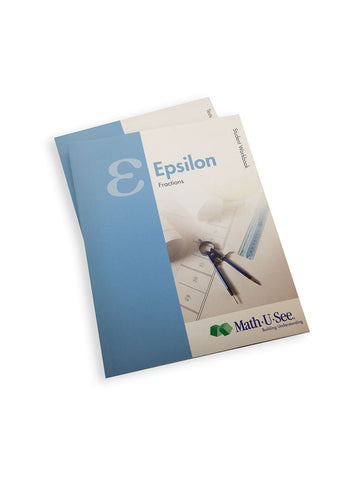# Math-U-See Epsilon Student Pack

• \$ 44.00

The Epsilon Student Pack contains the Student Workbook with lesson-by-lesson worksheets, systematic review pages, and Application and Enrichment pages as well as Epsilon Tests

Now that students have learned basic operations with whole numbers, Epsilon covers these same operations with fractions. Fractions are presented in an intuitive way with visual explanations of equivalent fractions, common denominators, and fractions and numbers larger than 1. Fractions and operations are illustrated using our proprietary Fraction Overlay manipulatives.